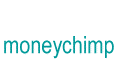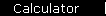# Basic Investment (Growth and Contributions)

A "basic investment" is one where you start with an initial principal, invest it at an annually compounded rate of return, and add equal contributions every year.

One thing we need to get straight from the beginning is the timing of the interest and contributions. We're going to assume that the balance of the account for any particular year includes the interest growth from last year, but does not yet include the new contribution. (This is the way most people do it, but sometimes you'll see an example that assumes a different schedule; they will disagree with our formula by the equivalent of one year's interest and/or one year's contribution).

We'll write c for the annual contribution; and to keep things cleaner we'll write "z" for (1 + r). Now we start writing down the account balance for the first few years:

 Year Balance Now P 1 (P + c)z 2 ((P + c)z + c)z

In other words, to go from one year's balance to the next, you add on the contribution c, then multiply by z to get the interest.

If you multiply these terms out, you'll start to see the pattern emerge:

 Year Balance Now P 1 Pz + cz 2 Pz2 + c(z + z2) Y PzY + c(z + z2 + . . . + zY)

The second part of that last line is just c times the sum of a geometric series. So you can boil the whole thing down to:

 1 Balance(n) = PzY + c[(zY + 1 - z)/(z - 1)]

Finally, write z out in terms of r, to get the formula we're looking for:

 2 Balance(Y)   =   P(1 + r)Y   +   c[ ((1 + r)Y + 1 - (1 + r)) / r ]

## Timing Issues

The formula above assumes contributions occur at the start of each year. If you want them to happen at the end instead, then you get one less interest period per contribution:

 Year Balance Now P 1 Pz + c 2 Pz2 + c(1 + z) Y PzY + c(1 + z + z2 + . . . + zY-1)

and the formula becomes:

 2a. Balance(Y)   =   P(1 + r)Y   +   c[ ((1 + r)Y - 1) / r ]

home  |  article  |  glossary  |  calculator  |  about us  |  booksIntroductionCompound InterestMore CompoundingPresent Value / CAGRComposite InvestmentsBond YieldGeometric SeriesGrowth + ContributionsAnnuityMortgageStock ValuationBooks & Links

 Also see the compound interest calculator.